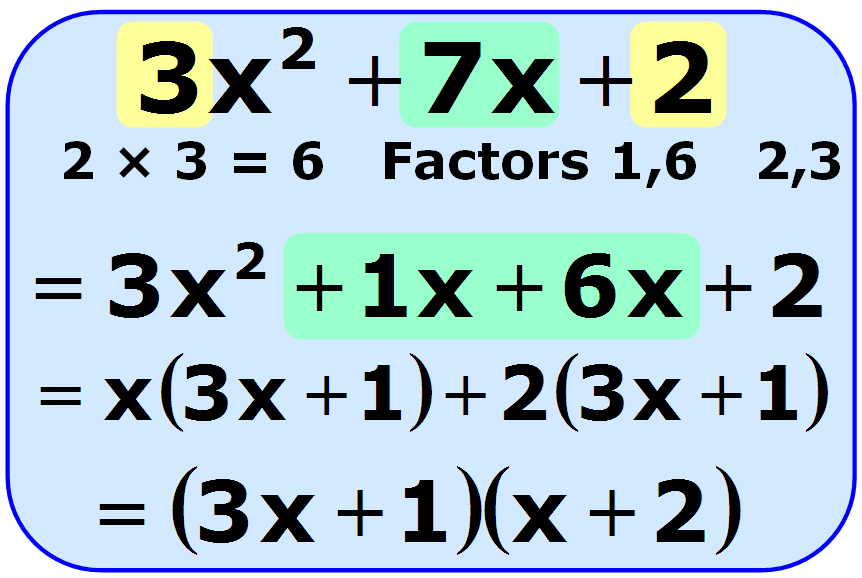# SOLVING QUADRATIC EQUATIONS USING FACTORISATION METHOD (VIDEO)Watch the video here on how to solve quadratic equations using factorisation method:

After watching the video, try and solve the following quadratic equations using factorisation method:

(1) x2 + 6x + 5  = 0                                          (2) x2 – 2x  – 3 = 0                                         (3) 2x2 – 5x  – 3 = 0                         (4) 2x2 – 3x – 20 = 0

(Photo Credit: maths.nayland.school.nz)      )